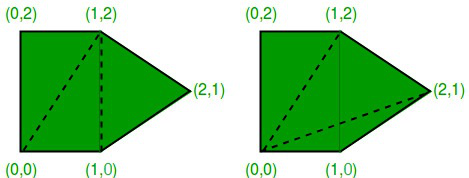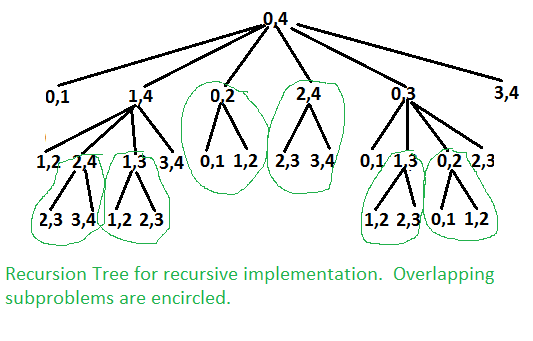Related Articles

# Minimum Cost Polygon Triangulation

• Difficulty Level : Hard
• Last Updated : 28 Jun, 2021

A triangulation of a convex polygon is formed by drawing diagonals between non-adjacent vertices (corners) such that the diagonals never intersect. The problem is to find the cost of triangulation with the minimum cost. The cost of a triangulation is sum of the weights of its component triangles. Weight of each triangle is its perimeter (sum of lengths of all sides)
See following example taken from this source.Two triangulations of the same convex pentagon. The triangulation on the left has a cost of 8 + 2√2 + 2√5 (approximately 15.30), the one on the right has a cost of 4 + 2√2 + 4√5 (approximately 15.77).

This problem has recursive substructure. The idea is to divide the polygon into three parts: a single triangle, the sub-polygon to the left, and the sub-polygon to the right. We try all possible divisions like this and find the one that minimizes the cost of the triangle plus the cost of the triangulation of the two sub-polygons.

```Let Minimum Cost of triangulation of vertices from i to j be minCost(i, j)
If j < i + 2 Then
minCost(i, j) = 0
Else
minCost(i, j) = Min { minCost(i, k) + minCost(k, j) + cost(i, k, j) }
Here k varies from 'i+1' to 'j-1'

Cost of a triangle formed by edges (i, j), (j, k) and (k, i) is
cost(i, j, k)  = dist(i, j) + dist(j, k) + dist(k, i)```

Following is implementation of above naive recursive formula.

## C++

 `// Recursive implementation for minimum cost convex polygon triangulation``#include ``#include ``#define MAX 1000000.0``using` `namespace` `std;` `// Structure of a point in 2D plane``struct` `Point``{``    ``int` `x, y;``};` `// Utility function to find minimum of two double values``double` `min(``double` `x, ``double` `y)``{``    ``return` `(x <= y)? x : y;``}` `// A utility function to find distance between two points in a plane``double` `dist(Point p1, Point p2)``{``    ``return` `sqrt``((p1.x - p2.x)*(p1.x - p2.x) +``                ``(p1.y - p2.y)*(p1.y - p2.y));``}` `// A utility function to find cost of a triangle. The cost is considered``// as perimeter (sum of lengths of all edges) of the triangle``double` `cost(Point points[], ``int` `i, ``int` `j, ``int` `k)``{``    ``Point p1 = points[i], p2 = points[j], p3 = points[k];``    ``return` `dist(p1, p2) + dist(p2, p3) + dist(p3, p1);``}` `// A recursive function to find minimum cost of polygon triangulation``// The polygon is represented by points[i..j].``double` `mTC(Point points[], ``int` `i, ``int` `j)``{``   ``// There must be at least three points between i and j``   ``// (including i and j)``   ``if` `(j < i+2)``      ``return` `0;` `   ``// Initialize result as infinite``   ``double` `res = MAX;` `   ``// Find minimum triangulation by considering all``   ``for` `(``int` `k=i+1; k

## Java

 `// Class to store a point in the Euclidean plane``class` `Point``{``  ``int` `x, y;``  ``public` `Point(``int` `x, ``int` `y)``  ``{``    ``this``.x = x;``    ``this``.y = y;``  ``}` `  ``// Utility function to return the distance between two``  ``// vertices in a 2-dimensional plane``  ``public` `double` `dist(Point p)``  ``{` `    ``// The distance between vertices `(x1, y1)` & `(x2,``    ``// y2)` is `√((x2 − x1) ^ 2 + (y2 − y1) ^ 2)```    ``return` `Math.sqrt((``this``.x - p.x) * (``this``.x - p.x)``                     ``+ (``this``.y - p.y) * (``this``.y - p.y));``  ``}``}` `class` `GFG``{` `  ``// Function to calculate the weight of optimal``  ``// triangulation of a convex polygon represented by a``  ``// given set of vertices `vertices[i..j]```  ``public` `static` `double` `MWT(Point[] vertices, ``int` `i, ``int` `j)``  ``{` `    ``// If the polygon has less than 3 vertices,``    ``// triangulation is not possible``    ``if` `(j < i + ``2``)``    ``{``      ``return` `0``;``    ``}` `    ``// keep track of the total weight of the minimum``    ``// weight triangulation of `MWT(i,j)```    ``double` `cost = Double.MAX_VALUE;` `    ``// consider all possible triangles `ikj` within the``    ``// polygon``    ``for` `(``int` `k = i + ``1``; k <= j - ``1``; k++)``    ``{` `      ``// The weight of a triangulation is the length``      ``// of perimeter of the triangle``      ``double` `weight = vertices[i].dist(vertices[j])``        ``+ vertices[j].dist(vertices[k])``        ``+ vertices[k].dist(vertices[i]);` `      ``// choose the vertex `k` that leads to the``      ``// minimum total weight``      ``cost = Double.min(cost,``                        ``weight + MWT(vertices, i, k)``                        ``+ MWT(vertices, k, j));``    ``}``    ``return` `cost;``  ``}` `  ``// Driver code``  ``public` `static` `void` `main(String[] args)``  ``{` `    ``// vertices are given in clockwise order``    ``Point[] vertices``      ``= { ``new` `Point(``0``, ``0``), ``new` `Point(``2``, ``0``),``         ``new` `Point(``2``, ``1``), ``new` `Point(``1``, ``2``),``         ``new` `Point(``0``, ``1``) };` `    ``System.out.println(MWT(vertices,``                           ``0``, vertices.length - ``1``));``  ``}``}` `// This code is contributed by Priiyadarshini Kumari`

## Python3

 `# Recursive implementation for minimum``# cost convex polygon triangulation``from` `math ``import` `sqrt``MAX` `=` `1000000.0` `# A utility function to find distance``# between two points in a plane``def` `dist(p1, p2):``    ``return` `sqrt((p1[``0``] ``-` `p2[``0``])``*``(p1[``0``] ``-` `p2[``0``]) ``+` `\``                ``(p1[``1``] ``-` `p2[``1``])``*``(p1[``1``] ``-` `p2[``1``]))` `# A utility function to find cost of``# a triangle. The cost is considered``# as perimeter (sum of lengths of all edges)``# of the triangle``def` `cost(points, i, j, k):``    ``p1 ``=` `points[i]``    ``p2 ``=` `points[j]``    ``p3 ``=` `points[k]``    ``return` `dist(p1, p2) ``+` `dist(p2, p3) ``+` `dist(p3, p1)`  `# A recursive function to find minimum``# cost of polygon triangulation``# The polygon is represented by points[i..j].``def` `mTC(points, i, j):``    ` `    ``# There must be at least three points between i and j``    ``# (including i and j)``    ``if` `(j < i ``+` `2``):``        ``return` `0``        ` `    ``# Initialize result as infinite``    ``res ``=` `MAX``    ` `    ``# Find minimum triangulation by considering all``    ``for` `k ``in` `range``(i ``+` `1``, j):``        ``res ``=` `min``(res, (mTC(points, i, k) ``+` `\``                        ``mTC(points, k, j) ``+` `\``                        ``cost(points, i, k, j)))``    ` `    ``return` `round``(res, ``4``)`  `# Driver code``points ``=` `[[``0``, ``0``], [``1``, ``0``], [``2``, ``1``], [``1``, ``2``], [``0``, ``2``]]``n ``=` `len``(points)``print``(mTC(points, ``0``, n``-``1``))` `# This code is contributed by SHUBHAMSINGH10`

Output:

`15.3006`

The above problem is similar to Matrix Chain Multiplication. The following is recursion tree for mTC(points[], 0, 4).It can be easily seen in the above recursion tree that the problem has many overlapping subproblems. Since the problem has both properties: Optimal Substructure and Overlapping Subproblems, it can be efficiently solved using dynamic programming.
Following is C++ implementation of dynamic programming solution.

## C

 `// A Dynamic Programming based program to find minimum cost of convex``// polygon triangulation``#include ``#include ``#define MAX 1000000.0``using` `namespace` `std;` `// Structure of a point in 2D plane``struct` `Point``{``    ``int` `x, y;``};` `// Utility function to find minimum of two double values``double` `min(``double` `x, ``double` `y)``{``    ``return` `(x <= y)? x : y;``}` `// A utility function to find distance between two points in a plane``double` `dist(Point p1, Point p2)``{``    ``return` `sqrt``((p1.x - p2.x)*(p1.x - p2.x) +``                ``(p1.y - p2.y)*(p1.y - p2.y));``}` `// A utility function to find cost of a triangle. The cost is considered``// as perimeter (sum of lengths of all edges) of the triangle``double` `cost(Point points[], ``int` `i, ``int` `j, ``int` `k)``{``    ``Point p1 = points[i], p2 = points[j], p3 = points[k];``    ``return` `dist(p1, p2) + dist(p2, p3) + dist(p3, p1);``}` `// A Dynamic programming based function to find minimum cost for convex``// polygon triangulation.``double` `mTCDP(Point points[], ``int` `n)``{``   ``// There must be at least 3 points to form a triangle``   ``if` `(n < 3)``      ``return` `0;` `   ``// table to store results of subproblems.  table[i][j] stores cost of``   ``// triangulation of points from i to j.  The entry table[n-1] stores``   ``// the final result.``   ``double` `table[n][n];` `   ``// Fill table using above recursive formula. Note that the table``   ``// is filled in diagonal fashion i.e., from diagonal elements to``   ``// table[n-1] which is the result.``   ``for` `(``int` `gap = 0; gap < n; gap++)``   ``{``      ``for` `(``int` `i = 0, j = gap; j < n; i++, j++)``      ``{``          ``if` `(j < i+2)``             ``table[i][j] = 0.0;``          ``else``          ``{``              ``table[i][j] = MAX;``              ``for` `(``int` `k = i+1; k < j; k++)``              ``{``                ``double` `val = table[i][k] + table[k][j] + cost(points,i,j,k);``                ``if` `(table[i][j] > val)``                     ``table[i][j] = val;``              ``}``          ``}``      ``}``   ``}``   ``return`  `table[n-1];``}` `// Driver program to test above functions``int` `main()``{``    ``Point points[] = {{0, 0}, {1, 0}, {2, 1}, {1, 2}, {0, 2}};``    ``int` `n = ``sizeof``(points)/``sizeof``(points);``    ``cout << mTCDP(points, n);``    ``return` `0;``}`

Output:

`15.3006`

Time complexity of the above dynamic programming solution is O(n3).
Please note that the above implementations assume that the points of covnvex polygon are given in order (either clockwise or anticlockwise)
Exercise:
Extend the above solution to print triangulation also. For the above example, the optimal triangulation is 0 3 4, 0 1 3, and 1 2 3.
Sources:
http://www.cs.utexas.edu/users/djimenez/utsa/cs3343/lecture12.html
http://www.cs.utoronto.ca/~heap/Courses/270F02/A4/chains/node2.html